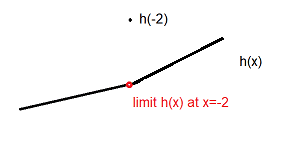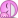# Limits on Composite Functions- Appears DNE but has a limit

• B
• opus

#### opus

Gold Member
Please see my attached image, which is a screenshot from Khan Academy on the limits of composite functions.

I just want to check if I'm understanding this correctly, particularly for #1, which has work shown on the picture.

Now my question:
We are taking the limit of a composition of functions, namely f(x) + g(x).
Now for the limit laws to work, as I understand, a limit has to exist with what you're starting with, and a limit has to exist for what you turn it into. In other words, a limit must exist in the RHS and LHS.
Now for problem 1, the limit of f(x) as x approaches -2 clearly does not exist. And the limit of g(x) as x approaches -2 does not exist. So how can we use the limit laws on this? A limit doesn't exist at the value that we are taking the limit of for each of the functions in the composition?

In the video, he goes on to take the limit of f(x) and g(x) as x approaches -2 from the left, and separately from the right. And these limits each sum to 4, so then it is said that the limit of the composition is 4.
I do understand this reasoning, but what isn't sitting right with me is the fact that the limits didn't exist at x=-2 in the first place, but by going at x=-2 from each side individually, the limit now exists.

#### Attachments

•Screen Shot 2018-09-09 at 2.07.33 PM.png
36.8 KB · Views: 1,991
Edit: I was using the term "composite" but what I actually went was "Combined".

We are taking the limit of a composition of functions, namely f(x) + g(x).
Now for the limit laws to work, as I understand, a limit has to exist with what you're starting with,...
Which is a function ##h\, : \,\mathbb{R} \longrightarrow \mathbb{R}## obtained as ##h(x) := \left( f(x)+g(x) \right)## - brackets first!
... and a limit has to exist for what you turn it into. In other words, a limit must exist in the RHS and LHS.
What do you mean by LHS and RHS? The limit and the ##4\,##?
Now for problem 1, the limit of f(x) as x approaches -2 clearly does not exist. And the limit of g(x) as x approaches -2 does not exist. So how can we use the limit laws on this? A limit doesn't exist at the value that we are taking the limit of for each of the functions in the composition?

In the video, he goes on to take the limit of f(x) and g(x) as x approaches -2 from the left, and separately from the right. And these limits each sum to 4, so then it is said that the limit of the composition is 4.
I do understand this reasoning, but what isn't sitting right with me is the fact that the limits didn't exist at x=-2 in the first place, but by going at x=-2 from each side individually, the limit now exists.
The limits of the component functions are irrelevant. Only if they existed, then we would have ##\lim h= \lim f +\lim g##. Here we are talking about ##h(x)##. Strictly speaking we would have to distinguish
$$\lim_{x \to -2 +0} h(x) = \lim_{x \to -2 +0}(f(-2)+g(-2))=3+1$$
and
$$\lim_{x \to -2 -0} h(x) = \lim_{x \to -2 -0}(f(-2)+g(-2))=1+3$$
In any case, we have to consider the function ##h(x)## which adds up differently from the right and from the left, but as the sums are equal, the limit exists.

##h(x)## is not continuous at ##x=-2##. If we approach its value from the left or from the right, we get the limit ##4##. But we have ##h(-2)=6##, so ##h(x)## around ##x=-2## is a line where the function value at ##x=-2## is two units above the rest.

Last edited:
What do you mean by LHS and RHS? The limit and the 44\,?
What is mean is the Right Hand Side of the Equation and the Left Hand Side of the equation. In other words, if I have the limit of an expression on the right hand side, and want to use the limit laws to break it up, a) a limit must exist for what we are starting with (on the LHS), and a limit must exist for each "piece" we break it up into (on the right hand side).

The limits of the component functions are irrelevant. Only if they existed, then we would have limh=limf+limg\lim h= \lim f +\lim g. Here we are talking about h(x)h(x).
Ok so since the limit of ##f(x)## as x approaches ##-2## doesn't exist, and the limit of ##g(x)## as x approaches ##-2## doesn't exist, we cannot say that the ##lim~h = lim~f + lim~g## because they don't individually have limits, so there are no limits to add?

By ##h(x)##, you are referring to the function that is the combination of ##f(x)+g(x)##?
And let me spell this out to see if I understand correctly:

We have ##\lim_{x \rightarrow -2} {f(x) + g(x)}##
##\lim_{x \rightarrow -2} {f(x)}## Does Not Exist
##\lim_{x \rightarrow -2} {g(x)}## Does Not Exist
However, this is irrelevant because we want the limit of h(x) which is their combination.
So what we can do is take ##\lim_{x \rightarrow -2^-} {f(x)} + \lim_{x \rightarrow -2^-} {g(x)} = L##
Then we can take ##\lim_{x \rightarrow -2^+} {f(x)} + \lim_{x \rightarrow -2^+} {g(x)} = M##
If ##L=M##, that means that as ##h(x)##, which is the combination of ##f## and ##g##, approaches ##-2## from the left and right, then ##\lim_{x \rightarrow -2}{h(x)}## EXISTS?

What is mean is the Right Hand Side of the Equation and the Left Hand Side of the equation. In other words, if I have the limit of an expression on the right hand side, and want to use the limit laws to break it up, a) a limit must exist for what we are starting with (on the LHS), and a limit must exist for each "piece" we break it up into (on the right hand side).
Yes, but without mention the equation you talk about, this is pretty senseless. All I saw was ##\lim_{x \to -2} (f(x)+g(x))=4## and it didn't appear as if you would talk about ##4##. There is simply no RHS to talk about!
Ok so since the limit of ##f(x)## as x approaches ##-2## doesn't exist, and the limit of ##g(x)## as x approaches ##-2## doesn't exist, we cannot say that the ##lim~h = lim~f + lim~g## because they don't individually have limits, so there are no limits to add?
Firstly, we have to distinguish right from left approaches here. Secondly, you are right. One of the two limits ##\lim f## and ##\lim g## doesn't exist on neither side. The formula is not applicable.
By ##h(x)##, you are referring to the function that is the combination of ##f(x)+g(x)##?
No. By ##x \mapsto h(x)## I was referring to exactly what I defined. I only used ##f(x)## and ##g(x)## for every single value of ##x##. I did not use the functions as such, neither did I compose anything. I picked values ##f(a)## and ##g(a)##, i.e. real numbers, added them - as numbers - and named the result ##h(a)##. As I did this for all values ##a##, I got a function ##a \mapsto h(a)##, only that I named my new variable ##x## instead of ##a##. But I did not use any property of ##f## or ##g## which says they are functions.
And let me spell this out to see if I understand correctly:
As long as you refuse to distinguish between left and right, any answer to this is risky, because it allows misunderstandings. What do you mean by "do not exist"? In our case both limits exist (left and right), but they are not the same, so the limit as such does not exist, correct, but this is for the technical requirement, that we only speak of a limit (if left and right aren't mentioned), if they coincide.
We have ##\lim_{x \rightarrow -2} {f(x) + g(x)}##
##\lim_{x \rightarrow -2} {f(x)}## Does Not Exist
##\lim_{x \rightarrow -2} {g(x)}## Does Not Exist
Yes, because left and right do not match. Otherwise they do exist.
However, this is irrelevant because we want the limit of h(x) which is their combination.
We want the limit of the expression in square brackets. Combination is unfortunate here, as it suggests we would need the two components. We only use them to define a sum, that's all.
So what we can do is take ##\lim_{x \rightarrow -2^-} {f(x)} + \lim_{x \rightarrow -2^-} {g(x)} = L##
Then we can take ##\lim_{x \rightarrow -2^+} {f(x)} + \lim_{x \rightarrow -2^+} {g(x)} = M##
If ##L=M##, that means that as ##h(x)##, which is the combination of ##f## and ##g##, approaches ##-2## from the left and right, then ##\lim_{x \rightarrow -2}{h(x)}## EXISTS?
Yes. Since a) the existence of those individual limits is given, so that the formula for addition is applicable in each individual case, and b) the technical requirement of being equal in order to speak of a limit is fulfilled.

Short:
##\lim_{x \to -2^+}f(x) \text{ defined }\; , \; \lim_{x \to -2^-}f(x) \text{ defined }\; , \;\lim_{x \to -2}f(x) \text{ undefined }##
##\lim_{x \to -2^+}g(x) \text{ defined }\; , \; \lim_{x \to -2^-}g(x) \text{ defined }\; , \;\lim_{x \to -2}g(x) \text{ undefined }##
##\lim_{x \to -2^+}h(x) \text{ defined }\; , \; \lim_{x \to -2^-}h(x) \text{ defined }\; , \;\lim_{x \to -2}h(x) \text{ defined }##
##\lim_{x \to -2} h(x) = 4 \neq 6 = h(-2)\; , \;h(x) \text{ is discontinuous }##

Last edited:
•opus
To summarize what @fresh_42 wrote in his short version:
##\lim_{x \to -2^-}f(x) = 1## and ##\lim_{x \to -2^+}f(x) = 3##
Therefore, ##\lim_{x \to -2}f(x)## does not exist.
Similarly, since the left- and right-hand limits for g(x) as x approaches -2 are different, ##\lim_{x \to -2}g(x)## also does not exist, so the two-sided limit of f(x) + g(x) doesn't exist as x approaches -2.

•opus
Here's a sketch, not exactly the actual values, i.e. I haven't checked the slope or so, but that's the crucial point here:#### Attachments

•opus
Similarly, since the left- and right-hand limits for g(x) as x approaches -2 are different, limx→−2g(x)limx→−2g(x)\lim_{x \to -2}g(x) also does not exist, so the two-sided limit of f(x) + g(x) doesn't exist as x approaches -2.
It seems that I was looking only at the consitutent functions f and g. It's very possible that lim(f + g) exists at a particular point, even though lim f and lim g don't exist at that point.

•opus
Thanks for the responses guys. Still trying to dissect this a bit. Going to take a mental breather from this and come back to it tonight for a proper reply.